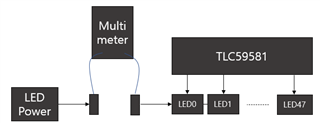If you have a related question, please click the "Ask a related question" button in the top right corner. The newly created question will be automatically linked to this question.

# TLC59581: How to select IREF Resistor

Part Number: TLC59581

Hi,

I am testing by applying TLC59581 to the new project.

Depending on the input data, LED control operates correctly.

I set the IREF resistor value by referring to item 9.3.4.1 of the datasheet.(example1)

I use one type of LED, and the target current is 20 mA.

The register setting value is as follows.

CCR, CCG, CCB : 511d

BC : 7h

IREF resistor : 1.209/20mA * 157.4 = 9.5K

However, measuring the current supplied to the LED consumes about 10 mA.

I want to target the maximum current of 20 mA and adjust the brightness through BC.

thanks.

• Hi Jansu,

If you want to change the Brightness, you can adjust both BC and CC code.

For example, the REF is set to 9.5k, please set the BC to 011(82.4) and set CC to 488, the LED current can be targeted to 10mA.

Best Regard

Monet Xu

• Hi Monet,

I know to set the target current through BC and CC register.

If REF is set to 9.5k, set the BC to 111 and set CC to 511,

The target current is 20 mA, but the actual measurement is about 10.8 mA. (total current(520)/LED channel(48))

If BC and CC are set as your answer, it will be measured as 7.7 mA.(total current(370)/LED channel(48))

Output data is the maximum value.

Led's maximum rated current specification is 20mA.

Is there something wrong with how I measure it?thanks

• Hi Kim,

since the channel's current is AC signal so the LED constant current should not calculate by total current and channel number. if you want to check the real current, you can use a oscilloscope.

BR

Monet Xu